170W Voltage Doubler in 23mm x 16.5mm Package

Linear Technology LTC7820

Ya Liu, Jian Li, Jeff Zhang and Brian Lin, Analog Devices

Design Note 571

Introduction

For high voltage input/output applications, inductorless, switched capacitor converters (charge pumps) significantly improve efficiency and reduce solution size over conventional inductor-based buck or boost topologies. By using a charge pump instead of an inductor, a “flying capacitor” is used to store and transfer the energy from input to output. The energy density of capacitors is much higher than inductors, improving power density by a factor of 10 using a charge pump. However, charge pumps have traditionally been limited to low power applications, due to the challenges presented in start-up, protection, gate drive and regulation.

The LTC7820 overcomes these problems, allowing high power density, high efficiency (to 99%) solutions. This fixed-ratio high voltage, high power switched capacitor controller includes four N-channel MOSFET gate drivers, for use with external power MOSFETs, to produce a voltage divider, doubler, or inverter: specifically, 2:1 step-down from inputs to 72 V, 1:2 step-up from input voltages to 36 V, or a 1:1 inverter from inputs to 36 V. Each power MOSFET is switched with 50% duty cycle at a constant preprogrammed switching frequency.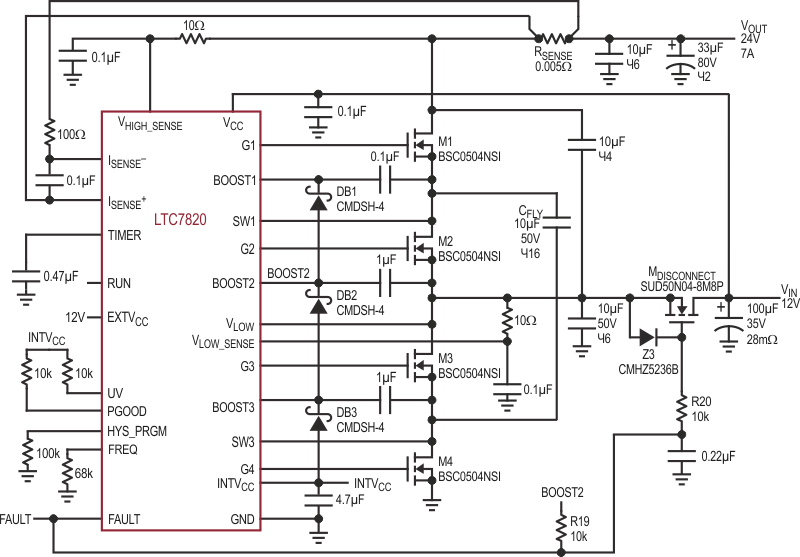Figure 1. A High Efficiency, High Power Density 12 V VIN to 24 V/7 A Voltage Doubler Using the LTC7820.

Figure 1 shows a 170 W output voltage doubler circuit featuring the LTC7820. The input voltage is 12 V and the output is 24 V at up to 7 A load, and a switching frequency of 500 kHz. Sixteen 10 μF ceramic capacitors (X7R, 1210 size) act as a flying capacitor to deliver output power. The approximate solution size is 23 mm × 16.5 mm × 5 mm as shown in Figure 2, and the power density is as high as 1500 W/in3.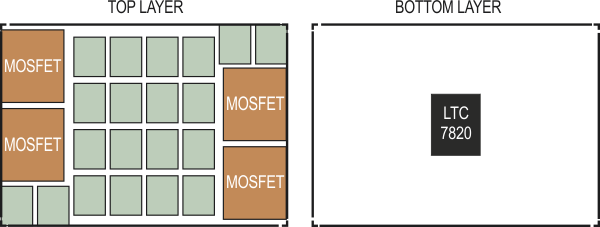Figure 2. Estimated Solution Size.

High Efficiency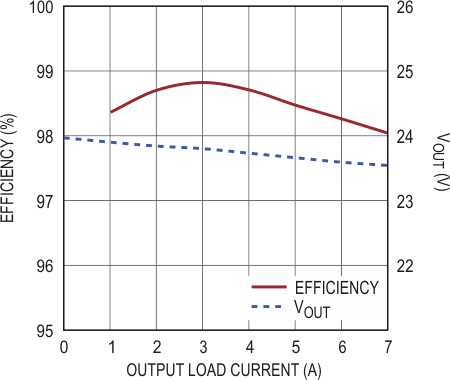Figure 3. Efficiency and Load Regulation of 12 V VIN to 24 V/7 A Voltage Doubler at 500 kHz fSW.

All four MOSFETs are soft switched because there is no inductor used in the circuit, greatly reducing switching-related losses. Furthermore, in a switched capacitor voltage doubler, low voltage rating MOSFETs can be used, significantly reducing conduction losses. As shown in Figure 3, the converter can achieve 98.8% peak efficiency and 98% efficiency at the full load. Power loss is balanced between the four switches, spreading thermal dissipation, and simplifying heat mitigation in a smart layout. The thermograph in Figure 4 shows a hot spot temperature rise of only 35 °C in free air in an ambient 23 °C.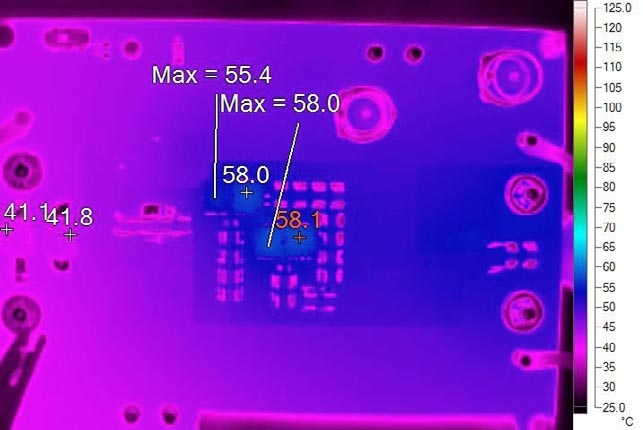Figure 4. Thermal Test at 12 V VIN, 24 V VOUT, 7 A Load, TA = 23 °C, Free Air.

The LTC7820-based voltage doubler is an open-loop converter, but the LTC7820’s high efficiency keeps regulation tight, as shown in Figure 3 – the output voltage drops only 0.43 V (1.8%) at full load.

Start-Up

In voltage doubler applications, LTC7820 can start-up without capacitor inrush charging current if the input voltage is ramping up slowly from zero. As long as the input voltage ramps up slow (in milliseconds), the output voltage can track the input voltage and the voltage difference between capacitors remains small, so there are no large inrush currents.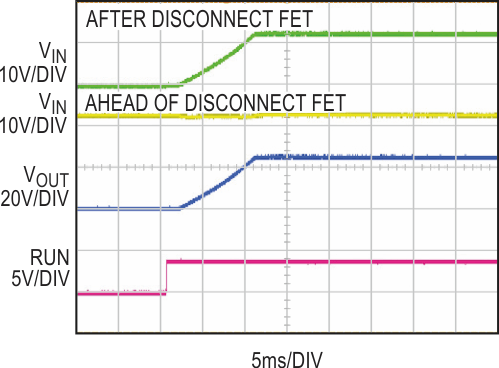Figure 5. Start-Up Waveform at 7 A Load.

Slew rate control of the input can be achieved by using a disconnect FET at input or using hot swap controllers, as shown in the typical application section in the LTC7820 data sheet. In Figure 1, a disconnect FET is used at the input. Unlike voltage divider solutions, the voltage doubler must start up from zero input voltage every time, but it can start up directly with heavy loads. Figure 5 shows the start-up at 7 A load.

Conclusion

The LTC7820 is a fixed ratio switched capacitor controller that drives external MOSFETs with built-in gate drivers, achieving very high efficiency (to 99%) and high power density. Robust protection features enable an LTC7820 switched capacitor converter to fit high voltage, high power applications such as bus converters, high power distributed power systems, communications systems and industrial applications.

cds.linear.com